# 【飞控】从零开始建模（三） - 控制分配浅析

### 0.如何得到电机转速

$$f=C_Tw^2$$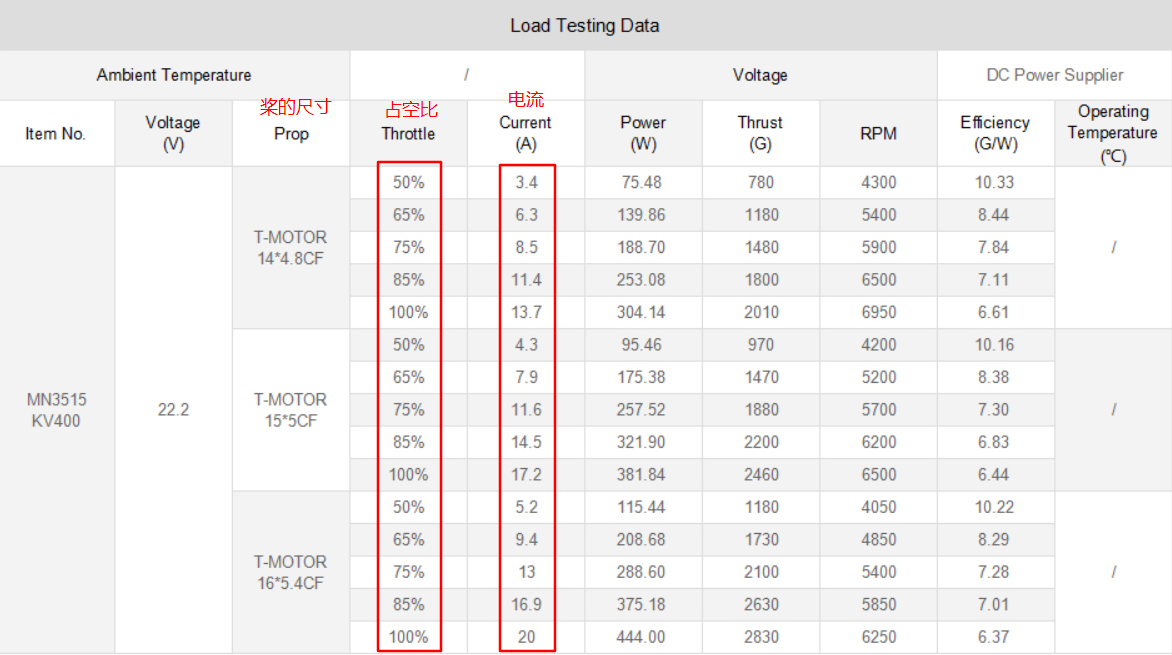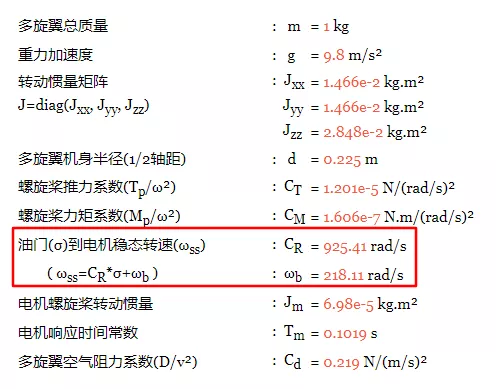### 1.控制器输出如何分配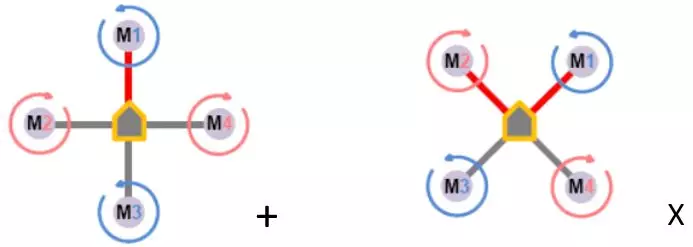[roll,pitch,yaw,thrust],那么电机应该有把与之相关的控制都叠加起来，所以

M1 = 0 - pitch + yaw + thrust
M2 = roll + 0 - yaw + thrust
M3 = 0 + pitch + yaw + thrust
M4 = - roll + 0 - yaw + thrust

### 2.更好的控制分配

M1 = 0 - pitch + yaw + 0.8 thrust
M2 = roll + 0 - yaw + 0.8
thrust
M3 = 0 + pitch + yaw + 0.8 thrust
M4 = - roll + 0 - yaw + 0.8
thrust

### 3.x型的控制分配系数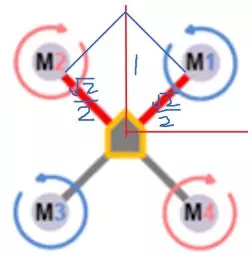M1 = - 0.707 roll - 0.707 pitch + yaw + 0.8 thrust
M2 = 0.707
roll - 0.707 pitch - yaw + 0.8 thrust
M3 = 0.707 roll + 0.707 pitch + yaw + 0.8 thrust
M4 = - 0.707
roll + 0.707 pitch - yaw + 0.8 thrust

### 4.APM源码

APM的相关源码在
libraries\AP_Motors\AP_Motors6DOF.cpp
libraries\AP_Motors\AP_MotorsMatrix.cpp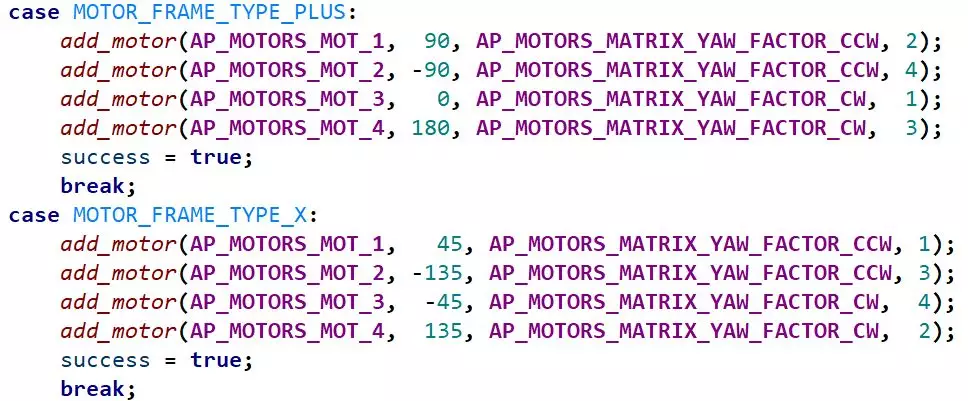### 5.背后的理论

$$f=c_T(w_1^{2}+w_2^{2}+w_3^{2}+w_4^{2})$$

roll,pitch,yaw三个通道分别对应x,y,z三轴的扭矩。(开源飞行器通常使用逆时针的顺序排列电机，可能会与书中不一样)

$$\begin{matrix} \tau_x=dc_T(w_2^{2}-w_4^{2})\\ \tau_y=dc_T(-w_1^{2}+w_3^{2})\\ \tau_z=c_M(w_1^{2}-w_2^{2}+w_3^{2}-w_4^{2})\\ \end{matrix}$$

c_T为升力系数，c_M为扭力系数，d为机体中心到电机的距离，w为电机转速。

$$\left[\begin{matrix} f \\\tau_x \\ \tau_y \\ \tau_z \end{matrix}\right]=\left[\begin{matrix} c_T & c_T & c_T &c_T\\ 0 & dc_T & 0 & -dc_T \\ -dc_T & 0 & dc_T & 0 \\ c_M & -c_M & c_M & -c_M \\ \end{matrix}\right]\left[\begin{matrix} w_1^{2} \\ w_2^{2} \\ w_3^{2} \\ w_4^{2} \end{matrix}\right]$$

$$\left[\begin{matrix} f \\\tau_x \\ \tau_y \\ \tau_z \end{matrix}\right]=M_4\left[\begin{matrix} w_1^{2} \\ w_2^{2} \\ w_3^{2} \\ w_4^{2} \end{matrix}\right]$$

$$M_4^{-1}\left[\begin{matrix} f \\\tau_x \\ \tau_y \\ \tau_z \end{matrix}\right]=\left[\begin{matrix} w_1^{2} \\ w_2^{2} \\ w_3^{2} \\ w_4^{2} \end{matrix}\right]$$

$$P_4=M_4^{-1}$$

$$\left[\begin{matrix} f \\\tau_x \\ \tau_y \\ \tau_z \end{matrix}\right]$$

### 6.参考资料